### 15.4.3.3 Philip Hall basis of a Lie algebra

Determining the basis of a Lie algebra may be a long and tedious process. The combinations of Lie brackets in Example 15.17 were given; however, it is not known in advance which ones will produce independent vector fields. Numerous Lie brackets may be needed, including some that are nested, such as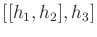. The maximum depth of nested Lie bracket operations is not known a priori. Therefore, a systematic search must be performed (this can in fact be modeled as a discrete planning problem) by starting with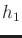,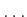,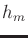and iteratively generating new, independent vector fields using Lie brackets.

One popular approach is to generate the Philip Hall basis (or P. Hall basis) of the Lie algebra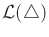. The construction of the basis essentially follows breadth-first search, in which the search depth is defined to be the number of nested levels of bracket operations. The order (or depth)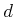of a Lie product is defined recursively as follows. For the base case, let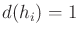for any of the system vector fields. For any Lie product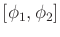, let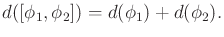(15.108)

Thus, the order is just the nesting depth (plus one) of the Lie bracket operations. For example,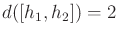and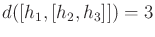.

In addition to standard breadth-first search, pruning should be automatically performed to ensure that the skew symmetry and Jacobi identities are always utilized to eliminate redundancy. A P. Hall basis is a sequence,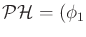,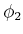,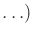, of Lie products for which:

1. The system vector fields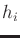are the first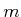elements of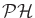.
2. If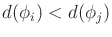, then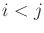.
3. Each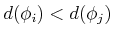if and only if: a)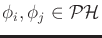and, and b) either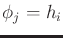for someor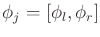for some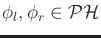such that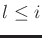.
It is shown in many algebra books (e.g., ) that this procedure results in a basis for the Lie algebra. Various algorithms for computing the basis are evaluated in .

Example 15..18 (P. Hall Basis Up to Depth Three)   The P. Hall basis sorts the Lie products into the following sequence, which is obtained up to depth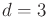:,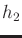,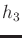,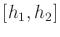,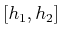,,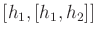,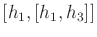,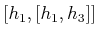,,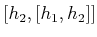,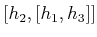,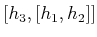,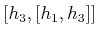.
So far, the only Lie product eliminated by the Jacobi identity isbecause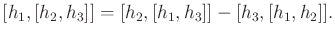(15.109)

Note that all of the Lie products given here may not be linearly independent vector fields. For a particular system, linear independence tests should be performed to delete any linearly dependent vector fields from the basis.When does the sequenceterminate? Recall that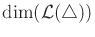can be no greater than, because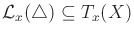. In other words, at every state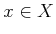, the number of possible independent velocity vectors is no more than the dimension of the tangent space at. Therefore,can be terminated onceindependent vector fields are obtained because there is no possibility of finding more. For some systems, there may be a depthafter which all Lie brackets are zero. Such systems are called nilpotent of order. This occurs, for example, if all components of all vector fields are polynomials. If the system is not nilpotent, then achieving termination may be difficult. It may be the case thatis strictly less than, but this is usually not known in advance. It is difficult to determine whether more Lie brackets are needed to increase the dimension or the limit has already been reached.

Steven M LaValle 2020-08-14